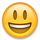edited April 2013
I was looking for a good way to "normalize" an angle in radians. By normalize I mean making sure the value is between 0 and 2 * pi (i.e. tau). I came across several implementations that simply didn't work right, so I made my own. These are pretty basic, but have been very useful.
``````/*
* Common.h
*
*  Created on: Apr 12, 2013
*      Author: epoulsen
*/

#ifndef COMMON_H_
#define COMMON_H_
#define _USE_MATH_DEFINES
#include <math.h>
#include "orx.h"

#define M_TAU 6.283185307
//
//  Python prototype
//
//    else:

}

return rad * (180 / M_PI);
}

inline float vec2rad(orxVECTOR & vec) {
}

}

#endif /* COMMON_H_ */
``````

• edited April 2013
Thanks for sharing that code snippet.

As fmod is famous to be a (relatively) expensive function, here's an alternative that should work just as well, without any conditional branching (which is an added bonus!):
``````float normalizeRadians(float rad)
{
float result;

// Scale the input by 1/Tau (ie. normalize)
result = rad * (1.0f / M_TAU);

// Apply a circular clamp to domain [0, 1[
result -= (float)((int)result - (int)(result < 0.0f));

// Scale back to original domain
result *= M_TAU;

// Done!
return result;
}
``````
Also, if you use orx, the whole vec2rad/rad2vec conversion already exists as part of the cartesian<->spherical translations:
``````// vec2rad
Cool, I've [strike]copied[/strike] implemented your normalizer